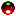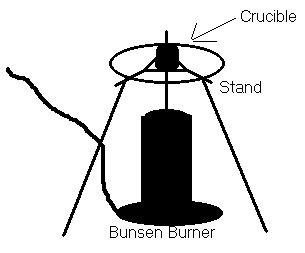CH - 120

Fall 2000

Definite Composition

Experiment #3

Submitted by:

Andrew J. Buettner

Partner:

none

Report Due: March 6, 2003Objective

The objective of this lab is to determine the maximum mass of sulfur that under specified conditions combines with a given mass of Copper.Apparatus

A) Equipment used

1) Crucible

2) Stand

3) Bunsen Burner

5) Sulfur in excess

6) Tongs

7) Scale

B) DiagramMethod

1) Mass the crucible.

2) Obtain about 1g of Copper.

3) Place the copper into the crucible and mass the result.

4) Add an amount of sulfur in excess.

5) Heat the crucible for about 10 minutes or until the sulfur has burnt off.

6) Mass the resulting remains.

7) Repeat steps 4 through 6 until all of the copper has reacted.Data

A) Table of masses

Initial

Heating 1

Heating 2

Mass

21.976g

22.110g

22.111g

Mass of copper:

.785g

.919g

.920g

B) Extra Data:

1) Mass of Crucible: 21.191gResults

* Note: All calculations are shown on attached sheets

A) Mass of sulfur that reacted with copper after heating 1: .934g

B) Mass of sulfur that reacted with copper after heating 2: .135g

C) Percentage mass of sulfur in total after heating one: 14.6%

D) Percentage mass of sulfur in total after heating two: 14.7%Answers to Lab Questions

1) Q: What is the uncertainty of each calculation in the results and data?

A: The results and readings have an error of +/- .0005

2) Q: Calculate the percentage of sulfur in CuS and Cu2S, and compare the values with the values obtained.

A: In CuS the percent mass of Sulfur is 33.538%, in Cu2S it is 20.147%. Both of these answers are greater than the percent obtained in the results.

3) Q: Explain how the following would affect the results:

a) Some of the copper reacted with oxygen to produce CuO.

b) Some of the copper reacted with the Oxygen to produce Cu2O.

c) Some of the copper did not react with the sulfur.

d) Some of the copper assumed to be pure was actually CuO.

A: a) The results would indicate a percent weight of sulfur lower than it actually was.

b) The results would indicate a percent weight of sulfur lower than it actually was.

c) The results would indicate a percent weight of sulfur lower than it actually was.

d) The results would indicate a percent that was higher then the actual percent was.

4) Q: If the experiment were somewhat deficient in copper from the expected amount, what impact would that have on the percent mass of sulfur?

A: The percent would be lower than expected.Conclusions

From this lab it is concluded that with .785g of what is assumed to be pure copper will, under the given conditions, react with .135g of sulfur to form combinations of CuS and Cu2S. However, these values are not totally accurate as the copper was not completely pure. This is evident from the deviation of 27.0% from that of pure Cu2S. To compensate for this difference, the copper must not have been pure and some of the copper did not fully react.Attachments

1) Original lab data.

2) Calculations worksheet.